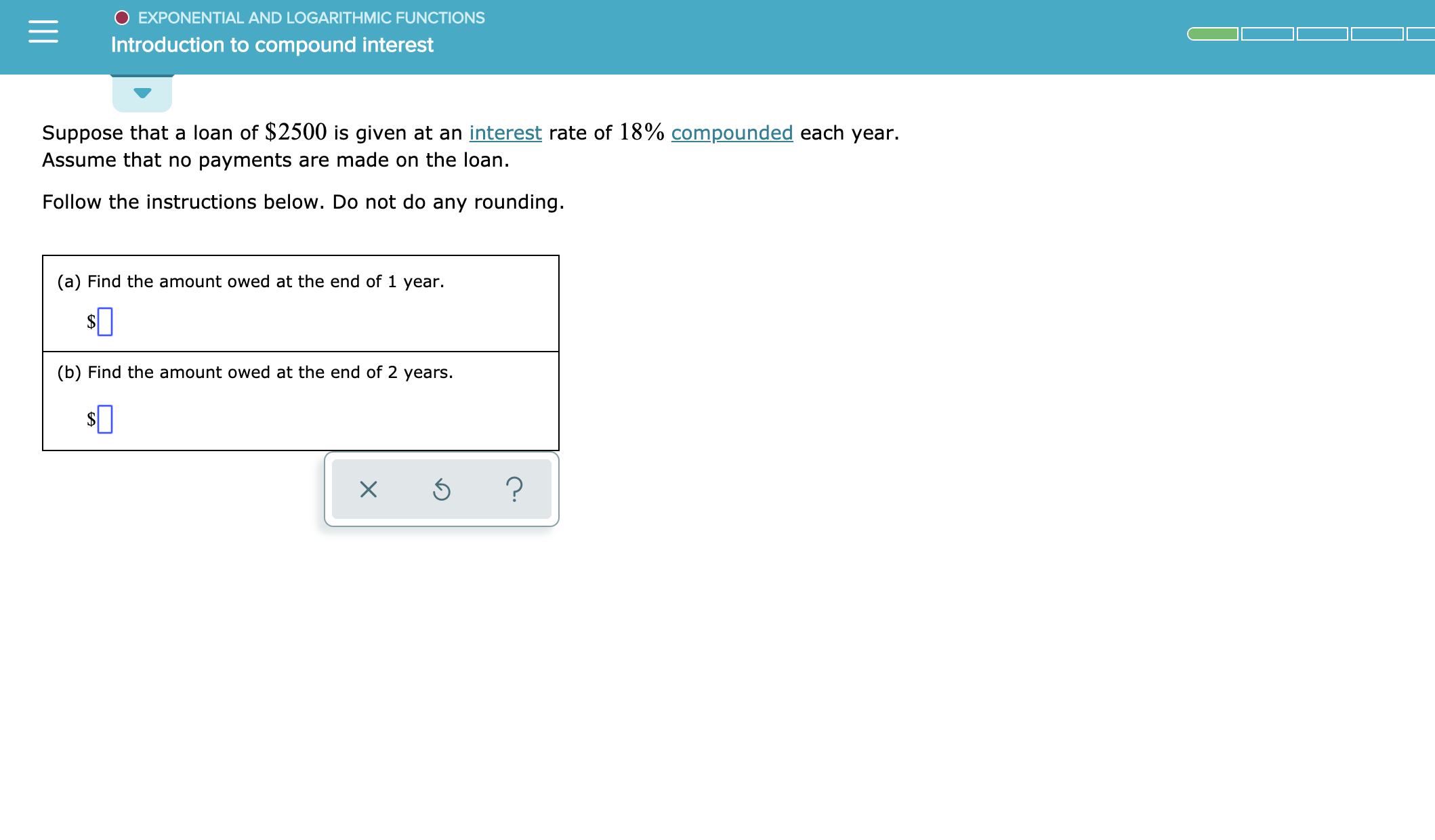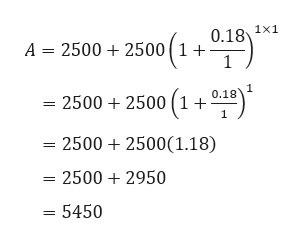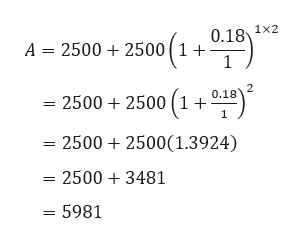# O EXPONENTIAL AND LOGARITHMIC FUNCTIONSIntroduction to compound interestSuppose that a loan of \$2500 is given at an interest rate of 18% compounded each year.Assume that no payments are made on the loan.Follow the instructions below. Do not do any rounding.(a) Find the amount owed at the end of 1 year.(b) Find the amount owed at the end of 2 years.?

Question

See attachedhelp_outlineImage TranscriptioncloseO EXPONENTIAL AND LOGARITHMIC FUNCTIONS Introduction to compound interest Suppose that a loan of \$2500 is given at an interest rate of 18% compounded each year. Assume that no payments are made on the loan. Follow the instructions below. Do not do any rounding. (a) Find the amount owed at the end of 1 year. (b) Find the amount owed at the end of 2 years. ? fullscreen
check_circleExpert Solution
Step 1

We know that,

Step 2

Part a

Here, P = \$2500, r = 18% = 0.18 and t = 1.

Since, it is compounded annually so n = 1.help_outlineImage Transcriptionclose0.18 1x1 A 2500 2500(1 +- 1 2500 2500 (1+ 0.18 = 25002500(1.18) - 25002950 = 5450 fullscreen
Step 3

Part b

Here, P = \$2500, r = 18% = 0.18 and t = 2.

Sinc...help_outlineImage Transcriptionclose0.18 1x2 A 2500 2500(1 +' 1 2 2500 2500 (1+ 0.18 2500 2500(1.3924) - 25003481 5981 fullscreen

### Want to see the full answer?

See Solution

#### Want to see this answer and more?

Solutions are written by subject experts who are available 24/7. Questions are typically answered within 1 hour*

See Solution
*Response times may vary by subject and question
Tagged in

### Other